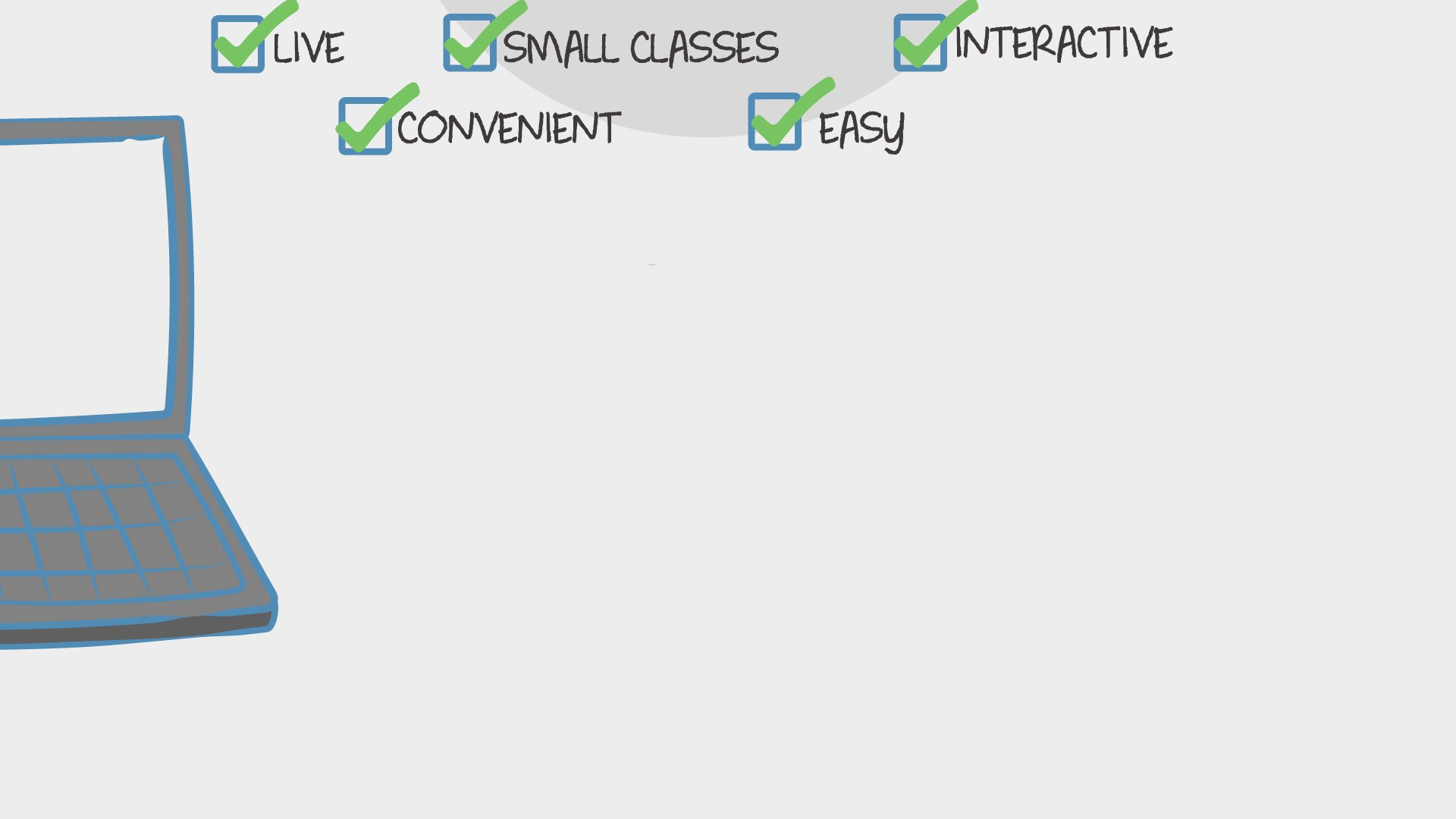Math

## Super Math Strategies: Multiplication Facts 0-12

In this 4-week class, students will learn several multiplication strategies to help them learn their multiplication facts 0-12. Using songs, games, and hands-on activities students will learn tips and tricks for multiplying.
41 total reviews for this teacher
2 reviews for this class
Completed by 6 learners
There are no upcoming classes.per class

over 4 weeks

year olds

#### 3-9

learners per class
per learner - per class

### How does a “Multi-Day” course work?

Meets multiple times at scheduled times
Live video chats, recorded and monitored for safety and quality
Discussions via classroom forum and private messages with the teacher
Great for engaging projects and interacting with diverse classmates from other states and countries### How Outschool Works### There are no open spots for this class.

You can request another time or scroll down to find more classes like this.

## Description

#### Class Experience

```CCSS.MATH.CONTENT.3.NBT.A.3
Multiply one-digit whole numbers by multiples of 10 in the range 10-90 (e.g., 9 × 80, 5 × 60) using strategies based on place value and properties of operations.

CCSS.MATH.CONTENT.3.OA.A.1
Interpret products of whole numbers, e.g., interpret 5 × 7 as the total number of objects in 5 groups of 7 objects each. For example, describe a context in which a total number of objects can be expressed as 5 × 7.

CCSS.MATH.CONTENT.3.OA.A.3
Use multiplication and division within 100 to solve word problems in situations involving equal groups, arrays, and measurement quantities, e.g., by using drawings and equations with a symbol for the unknown number to represent the problem.

CCSS.MATH.CONTENT.3.OA.A.4
Determine the unknown whole number in a multiplication or division equation relating three whole numbers. For example, determine the unknown number that makes the equation true in each of the equations 8 × ? = 48, 5 = _ ÷ 3, 6 × 6 = ?

CCSS.MATH.CONTENT.3.OA.B.5
Apply properties of operations as strategies to multiply and divide.2 Examples: If 6 × 4 = 24 is known, then 4 × 6 = 24 is also known. (Commutative property of multiplication.) 3 × 5 × 2 can be found by 3 × 5 = 15, then 15 × 2 = 30, or by 5 × 2 = 10, then 3 × 10 = 30. (Associative property of multiplication.) Knowing that 8 × 5 = 40 and 8 × 2 = 16, one can find 8 × 7 as 8 × (5 + 2) = (8 × 5) + (8 × 2) = 40 + 16 = 56. (Distributive property.)

CCSS.MATH.CONTENT.3.OA.C.7
Fluently multiply and divide within 100, using strategies such as the relationship between multiplication and division (e.g., knowing that 8 × 5 = 40, one knows 40 ÷ 5 = 8) or properties of operations. By the end of Grade 3, know from memory all products of two one-digit numbers

```
`I have taught 3rd or 4th grade for 17 of my 18 years, so I have taught hundreds of students how to learn their facts successfully.`
`There will be optional homework in the way of worksheets, online games, and fact practice. We will go over worksheets together in class.`
```Multiplication Toolbox
dice
paper
pencil
cereal, blocks, beans, buttons or paperclips for manipulatives
playdoh
crayons
paperclip for spinner
a character game piece (optional)
*you will need to print out several handouts```
`In addition to the Outschool classroom, this class uses:Nearpod`
`1 hour 40 minutes per week in class, and an estimated 0 - 1 hours per week outside of class.`
`It would be beneficial for your child if you could 3 hole punch their hand-outs and put them in a folder.  It will help keep them organized! The worksheets, activities and games for homework will be optional, but will have a great impact on your student's fact fluency.`

## Teacher

`Hi, friends! Welcome to my class! My courses will be in all elementary academic areas along with some crafting classes.  I love teaching! I come to you with 18 years of teaching experience and have taught all subjects in grades PK-4.  I earned my... `